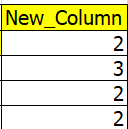cancel
Showing results for
Did you mean:Frequent Visitor

## Replace values with values in other column

table exampleI need thisThe DAX I wrote is

New_Column = CALCULATE(MIN('table'[Sup_group]),FILTER(ALL('table'),
'table'[province] = EARLIER('table'[province]) &&
'Stable'[Quarter] = "Q1"))

The result isBut I don't want this kind of result, I want like the first picture.

thank you.

1 ACCEPTED SOLUTIONSolution Sage

``​Column = CALCULATE(MIN([Sup_group]),ALLEXCEPT('Table','Table'[Group]))``

4 REPLIES 4Solution Sage

``​Column = CALCULATE(MIN([Sup_group]),ALLEXCEPT('Table','Table'[Group]))``Frequent Visitor

Oh, I can do it, just like you let me try. 👏Frequent Visitor

thank you, I tried to follow you result iswhich i want like thisSolution Sage

You are doing something wrong, look at my file
https://dropmefiles.com/XruU9